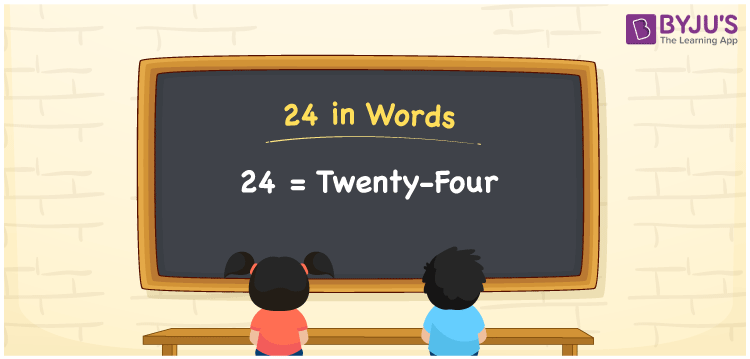# 24 in Words

24 in words is written as “Twenty-four”. In Mathematics, 24 is a natural number that is used to represent a value. To express the number we use English alphabets and write them in words. Hence, 24 is given by Twenty-four in English. For example, Mike gave his friend Rs.24 or Mike gave his friend Rupees Twenty Four. Learn more about Numbers In Words at BYJU’S.

 24 in Words Twenty-four Twenty-four in Numbers 24

## 24 in English Words

24 in Words using English alphabets is expressed as given below:## How to Write 24 in Words?

To write the number 24 in words, a place value chart can be used, where the position of each digit is recognised. Since 24 is a two-digit number, therefore, we have two place values here, i.e., ones and tens.

 Tens Ones 2 4

From the above table, we can see, 2 is at tens place and 4 is at ones place. We need to read the number from right to left, in the table. Thus, together we can write the number as Twenty-four.

### Expanded Form of 24

We can write the expanded form as:

2 × Ten + 4 × One

= 2 × 10 + 4 × 1

= 24

= Twenty-four

24 is a whole number that is succeeded by 23 and preceded by 25. Find more about the number 24 below:

• 24 in Words – Twenty-four
• Is 24 an odd number? – No
• Is 24 an even number? – Yes
• Is 24 a perfect square number? – No
• Is 24 a perfect cube number? – No
• Is 24 a prime number? – No
• Is 24 a composite number? – Yes

## Frequently Asked Questions on 24 in words

Q1

### What is 24 in words?

24 in words is given by Twenty-four.
Q2

### What is the rule to write 24 in words?

24 in words is written based on the place values of each digit. In 24, 2 is at tens place and 4 is at units place. Thus, twenty plus four is read as Twenty-four.
Q3

### How to write 24 in English words?

24 in English is written as Twenty-four